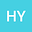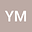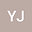Optimal time decay rates of solutions for the 2D generalized magneto-micropolar equations
•••Yiqiu Mao
Shenzhen University
Author Profileyan JIA
Shenzhen University
Author Profile#### Peer review status:UNDER REVIEW

24 Feb 2020Submitted to Mathematical Methods in the Applied Sciences
28 Feb 2020Assigned to Editor
28 Feb 2020Submission Checks Completed
29 Feb 2020Reviewer(s) Assigned
03 Jul 2020Review(s) Completed, Editorial Evaluation Pending

## Abstract

This study is concerned with the optimal time rates of weak solutions for the 2D magneto-micropolar equations with only micro-rotational dissipation and magnetic diffusion. Due to some new observations, we obtain the optimal time decay rates of weak solutions $\|\nabla u(t)\|_{L^2}+\|\nabla w(t)\|_{L^2}\le C(1+t)^{-2}$ and $\|\nabla b(t)\|_{L^{p}}\le C(1+t)^{-\frac12-(1-\frac1p)}$ with $p\in[2,\infty)$.